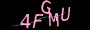×

# 收藏JavaScript中一些常用的代码性能优化技术【上篇】### 1.三元运算符

```const x = 20;
let answer;

if (x > 10) {
answer = "greater than 10";
} else {
answer =  "less than 10";
}```

`const answer = x > 10 ? "greater than 10" : "less than 10";`

`const answer = x > 10 ? "greater than 10" : x < 5 ? "less than 5" : "between 5 and 10";`

### 2.短路评估速记

```if (variable1 !== null || variable1 !== undefined || variable1 !== '') {
let variable2 = variable1;
}```

`const variable2 = variable1  || 'new';`

```let variable1;
let variable2 = variable1  || 'bar';
console.log(variable2 === 'bar'); // prints true

variable1 = 'foo';
variable2 = variable1  || 'bar';
console.log(variable2); // prints foo```

### 3.声明变量速记

```let x;
let y;
let z = 3;```

`let x, y, z=3;`

### 4.如果存在速记

`if (likeJavaScript === true)`

`if (likeJavaScript)`

```let a;
if ( a !== true ) {
// do something...
}```

```let a;
if ( !a ) {
// do something...
}```

### 5. JavaScript For循环速记

```const fruits = ['mango', 'peach', 'banana'];
for (let i = 0; i < fruits.length; i++)```

`for (let fruit of fruits)`

`for (let index in fruits)`

```const obj = {continent: 'Africa', country: 'Kenya', city: 'Nairobi'}
for (let key in obj)
console.log(key) // output: continent, country, city```

Array.forEach的简写：

```function logArrayElements(element, index, array) {
console.log("a[" + index + "] = " + element);
}
[2, 5, 9].forEach(logArrayElements);
// a = 2
// a = 5
// a = 9```

### 6.短路评估

```let dbHost;if (process.env.DB_HOST) {
dbHost = process.env.DB_HOST;
}else {
dbHost = 'localhost';
}```

`const dbHost = process.env.DB_HOST || 'localhost';`

### 7.小数基指数

`for (let i = 0; i < 10000; i++) {}`

```for (let i = 0; i < 1e7; i++) {}

// All the below will evaluate to true
1e0 === 1;
1e1 === 10;
1e2 === 100;
1e3 === 1000;
1e4 === 10000;
1e5 === 100000;```

### 8.对象属性速记

```const x = 1920,
y = 1080;
const obj = { x:x, y:y };```

`const obj = { x, y };`

### 9.箭头功能速记

```function sayHello(name) {
console.log('Hello', name);
}

setTimeout(function() {
console.log('Loaded')
}, 2000);

list.forEach(function(item) {
console.log(item);
});```

```sayHello = name => console.log('Hello', name);
setTimeout(() => console.log('Loaded'), 2000);
list.forEach(item => console.log(item));```

### 10.隐式回报速记

Return是我们经常用来返回函数最终结果的关键字。带有单个语句的箭头函数将隐式返回其评估结果（该函数必须省略花括号（`{}`）才能省略return关键字）。

```function calcCircumference(diameter) {
return Math.PI * diameter
}```

```calcCircumference = diameter => (
Math.PI * diameter;
)```

### 11.默认参数值

```function volume(l, w, h) {
if (w === undefined)
w = 3;
if (h === undefined)
h = 4;
return l * w * h;
}```

```volume = (l, w = 3, h = 4 ) => (l * w * h);
volume(2) //output: 24```

### 12.模板文字

```const welcome = 'You have logged in as ' + first + ' ' + last + '.'
const db = 'http://' + host + ':' + port + '/' + database;```

```const welcome = `You have logged in as \${first} \${last}`;
const db = `http://\${host}:\${port}/\${database}`;```

### 总结

https://www.jiangweishan.com/article/jsshoucang0931820938120983.html

### 网友评论文明上网理性发言已有0人参与### 最新留言

•### qianduan

这个跟H5做的很像，看上去用react实现起来要简单些。...

•### qianduan

YYDS的文章，收藏了。...

•### 访客

红红火火恍恍惚惚...

•### 跨境电商运营

谢谢站长的文章已经解决问题了...

•### 跨境电商运营

非常不错的文章下次还会再来！...

•### Web前端之家

可以的，有时间会发些关于SEO相关的文章~...

•### s4f

SEO很难啊，小编多发点类似文章吧！...

•### Web前端之家

应该没什么问题吧，等待官宣，毕竟还没正式公开这个版本。...

Copyright © 2021 Web前端之家(www.jiangweishan.com) 版权所有 All Rights Reserved.